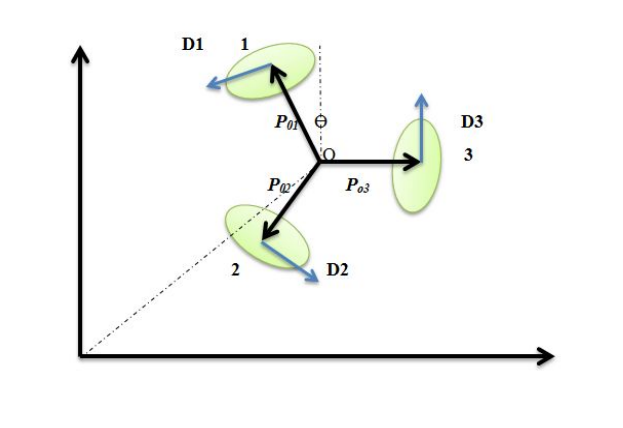A robot that uses omni-wheels can move in any direction, at any angle, without rotating beforehand. Such robots called holonomic robots can spin while translating forward at the same heading. A holonomic robot is one which is able to move instantaneously in any direction in the space of its degrees of freedom.
So how does a robot that can move omni-directionally work? The trick is that it uses special omni wheels as shown in fig 1. The omni-wheel is not actually just a single wheel, but many wheels in one. There is the big core wheel, and along the peripheral there are many additional small wheels that have the axis perpendicular to the axis of the core wheel. The core wheel can rotate around its axis like any normal wheel, but now that there are additional wheels perpendicular to it, the core wheel can also move parallel to it’s own axis.

### Holonomic and Non-Holonomic System

There are only two types of mobile robots, holonomic robots and non-holonomic robots. If the controllable degree of freedom is less than the total degrees of freedom, then it is known as non-Holonomic drive. A car has three degrees of freedom; i.e. its position in two axes and its orientation. However, there are only two controllable degrees of freedom which are acceleration (or braking) and turning angle of steering wheel. This makes it difficult for the driver to turn the car in any direction (unless the car skids or slides).Non-holonomic robots cannot instantaneously move in any direction, such as a car. This type of robot has to perform a set of motions to change heading. For example, if you want your car to move sideways, you must perform a complex ‘parallel parking’ motion. For the car to turn, you must rotate the wheels and drive forward.

A holonomic robot however can instantaneously move in any direction. It does not need to do any complex motions to achieve a particular heading. This type of robot would have 2 degrees of freedom in that it can move in both the X and Y plane freely. For e.g., the 3­-wheel omnidirectional robot has three differential degrees of freedom. Such a robot has no kinematic constraints, it is able to independently set all the three pose variables: x, y, θ. Holonomic refers to the relationship between controllable and total degrees of freedom of a robot. If the controllable degree of freedom is equal to total degrees of freedom, then the robot is said to be Holonomic.

### 3-Wheel Omnidirectional Robot Kinematics

The way in which the individual parts of a robot can move with respect to each other and the environment is called the ​kinematics of the robot. The forward kinematics specifies which direction the robot will drive in (linear velocities $$\dot{x}$$ and $$\dot{y}$$) and what its rotational velocity $$\dot{θ}$$ is based on the given individual wheel velocities. The inverse kinematics is a matrix formula that specifies the required individual wheel speeds for given desired linear and angular velocity ($$\dot{x}$$ , $$\dot{y}$$, $$\dot{θ}$$) and can be derived by inverting the matrix of the forward kinematics.

Holonomic robot design consisting of three Swedish $$90^{\circ}$$­ omnidirectional wheels placed 120 degrees apart is shown in Figure 3.
Figure 4 shows the geometric diagram of holonomic robot used to find its kinematic model. In the figure, O is the robot center of mass, $$P_​o$$ is defined as the vector connecting O to the origin and $$D​_i$$ is the drive direction vector of each wheel. The unitary rotation matrix R(θ) is defined as:

\begin{align*} & R(\theta)={ \begin{pmatrix} \cos\theta & -\sin\theta \\ \sin\theta & \cos\theta \end{pmatrix} }\\ & where, \theta\ is\ rotation\ angle\ in\ counter\ clockwise\ direction. \end{align*}

$$P_o$$ denotes the position of center of mass with respect to the world frame i.e. $$P_o = {[x\ y]}^T$$. The position $${[x_i\ \ y_i]}^T$$ of each wheel can be given with respect to the center of mass of the robot, i.e., for i=1, 2, 3.

\begin{align*} & P_{oi}= \begin{bmatrix} x_i\\ y_i \end{bmatrix} =R(\theta)L \begin{bmatrix} 0\\ 1 \end{bmatrix}\\ & where\ L\ is\ the\ distance\ of\ wheels\ from\ the\ robot\ center\ of\ mass\ (O). \end{align*}Fig 4. Kinematic Analysis of Holonomic Robot

$$P_{o1},P_{o2}$$ and $$P_{o3}$$ with respect to the local co-ordinates centered at the robot center of mass are given as:

\begin{align*} & P_{o1}=L \begin{bmatrix} 0\\ 1 \end{bmatrix}\\ & P_{o2}=R\left(\frac{2\pi}{3}\right)*P_{o1} =\frac{L}{2}\begin{bmatrix} -1\\ -\sqrt3 \end{bmatrix}\\ & P_{o3}=R\left(\frac{4\pi}{3}\right)*P_{o1} =\frac{L}{2}\begin{bmatrix} \sqrt3\\ -1 \end{bmatrix} \end{align*}

The drive directions $$D_i$$ of each wheel can be obtained by

\begin{align*} & D_{i}=\frac{1}{L}R\left(\frac{\pi}{2}\right) P_{oi},i=1,2,3 \end{align*}

which yields

\begin{align*} & D_{1}=\begin{bmatrix} -1\\ 0 \end{bmatrix}\\ & D_{2}=\frac{1}{2}\begin{bmatrix} \sqrt3\\ -1 \end{bmatrix}\\ & D_{3}=\frac{1}{2}\begin{bmatrix} 1\\ \sqrt3 \end{bmatrix} \end{align*}

The position and velocity of each wheel with respect to the world frame are then expressed by, for i = 1, 2, 3

\begin{align*} & R_i=P_o+R\left(\theta+\frac{2\pi}{3}(i-1)\right) P_{oi}\\ & v_i=\dot{P_o}+\dot{R}\left(\theta+\frac{2\pi}{3}(i-1)\right)P_{oi} \end{align*}

Translational velocity of each wheel is obtained as:

\begin{align*} & V_i={v_i}^T\left(R\left(\theta+\frac{2\pi}{3}(i-1)\right)D_{i}\right) \end{align*}

This gives

\begin{align*} & V_1=-\cos(\theta)\dot{x}-\sin(\theta)\dot{y}+L\dot{\theta}\\ & V_2=\cos\left(\frac{\pi}{3}-\theta \right)\dot{x}-\sin\left(\frac{\pi}{3}-\theta \right)\dot{y}+L\dot{\theta}\\ & V_3=\cos\left(\frac{\pi}{3}+\theta \right)\dot{x}+\sin\left(\frac{\pi}{3}+\theta \right)\dot{y}+L\dot{\theta}\\ \end{align*}

which can be written in matrix form as:

\begin{align*} & \begin{bmatrix} V_1\\ V_2\\ V_3 \end{bmatrix} =P(\theta) \begin{bmatrix} \dot{x}\\ \dot{y}\\ \dot{\theta} \end{bmatrix} \\ \\ & where\\ & P(\theta)= \begin{bmatrix} -\cos(\theta) & -\sin(\theta) & L\\ \cos\left(\frac{\pi}{3}-\theta \right) & \sin\left(\frac{\pi}{3}-\theta \right) & L\\ \cos\left(\frac{\pi}{3}+\theta \right) & \sin\left(\frac{\pi}{3}+\theta \right) & L \end{bmatrix} \end{align*}

is the inverse kinematics matrix. Matrix P(θ) is always singular for any value of θ. Thus,

\begin{align*} & P^{-1}(\theta)= \begin{bmatrix} -\frac{2}{3}\cos(\theta) & \frac{2}{3}\cos\left(\frac{\pi}{3}-\theta \right)& \frac{2}{3}\cos\left(\frac{\pi}{3}+\theta \right)\\ \frac{-2}{3}\sin(\theta) & \frac{-2}{3}\sin\left(\frac{\pi}{3}-\theta \right) & \frac{2}{3}\sin\left(\frac{\pi}{3}+\theta \right)\\ \frac{1}{3L} & \frac{1}{3L} & \frac{1}{3L} \end{bmatrix} \end{align*}

is the forward kinematics matrix which satisfies

\begin{align*} \begin{bmatrix} \dot{x}\\ \dot{y}\\ \dot{\theta} \end{bmatrix} =P^{-1}(\theta) & \begin{bmatrix} V_1\\ V_2\\ V_3 \end{bmatrix} \end{align*}
1. “Robot Platform-Holonomic vs. Non-Holonomic.” RSS. N.p., n.d. Web. 23 Sept. 2016.
2. “How to Build a Robot Tutorials - Society of Robots.” How to Build a Robot Tutorials - Society of Robots. N.p., n.d. Web. 23 Sept. 2016.
3. C.-C. Tsai, L.-B. Jiang, T.-Y. Wang and T.-S. Wang, “Kinematics Control of an Omnidirectional Mobile Robot,” in CACS Automatic Control Conference, 2005. -[pdf]